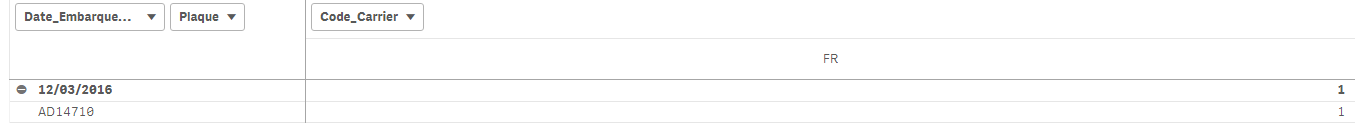# New to Qlik Sense

If you’re new to Qlik Sense, start with this Discussion Board and get up-to-speed quickly.

Announcements
Our May 2021 end-to-end product release from Data Integration to Data Analytics is out! READ DETAILS
cancel
Showing results for
Search instead for
Did you mean:Partner

## Transform a set expression to an expression in the script

Hi all (stalwar1‌),

Here is my demand,

I have a list of dates (date_embarquement), and a list of plaques (plaque):

for each date and plaque: we should only have ONE code_carrier.

In the source, we could find for Code_Ligne = MAR, in a date_embarquement and a plaque something like this:which is false since It's the same folder (dossier).

Here's the original expression:  count({<Code_Ligne={'MAR'}>}Dossier)

I changed it like this:

count({<Code_Ligne={'MAR'},

Dossier={"=Count(Aggr((Code_Carrier&Plaque),Date_Embarquement,Plaque))=1"}>}Dossier)

and the result is: (I think It's a success):Now, the question is; how to implement the previous expression in the script to force it to only bring one code_carrier per date_embarqueent and Plaque for code ligne maroc?

The script is as follow:

LOAD

Dossier,

"%Date ID",

Code_Activite,

Designation_Activite,

Sens,

Filiale_Creation_Dossier,

"Filiale Origine",

"Pays Fililale Origine",

"Filiale Destination",

Code_Ligne,

Num_Voyage,

Date_Dossier,

Date_Embarquement,

Date_Debarquement,

Groupe_Complet_Vide,

Plaque,

Parc,

Type_Materiel,

Compagnie,

Remorque_Parc_HorsParc,

Voyage_Ligne,

Code_Carrier,

Pays,

PAYS2,

Charge_Vide

FROM [lib://qvd/final_16-03-017.qvd]

(qvd)

Where Code_Ligne = 'MAR';

You'll find attached the 2 Qvds I'm working with : (calendar and final)

Thank you,

1 Solution

Accepted SolutionsPartner
Author

I found a solution.

29 RepliesPartner
Author

I found a solution.Partner
Author

stalwar1‌, can you help please?MVP

I thought you already found a solution to this?Partner
Author

Yes, but I have found yet another problem in my first expression;

Let me do a recap:

As I already said in the first msg above; I have to count (dossier) for each date_embarquement/plaque only in one carrier :

count({<Code_Ligne={'MAR'}

,Groupe_Complet_Vide={c,C,G}

,Dossier = {"=Count(Aggr((Code_Carrier&Plaque),Date_Embarquement,Plaque))=1"}>} Dossier)

Now, the problem is that I work with variables to do time based analytics (yearly, monthly etc)

To do so, I have to select a Year, a timeframe and so on :

It all works fine in the present period, BUT in the PREVIOUS one; it does not and I know why:

Here is the situation:

My expression for the previous period :

count({<\$(vSetPeriodPrev),Code_Ligne={'MAR'}

,Groupe_Complet_Vide={c,C,G}

,Dossier = {"=Count(Aggr((Code_Carrier&Plaque),Date_Embarquement,Plaque))=1"}>} Dossier)

Let's assume we selected yearly: the vSetPeriodPrev variable will call the YearlyPrev Variable which is like this:

[Calendar date]=,[Year of date]=,[Month of date]=,

[Year of date]={"\$(=max([Year of date])-\$1)"}

Now, the problem is : In my expression, for the previous period; when I select year of date=2017,

I want my expression to count code_carrier&plaque by date_embarquement in the year 2016 (which is the previous year) !

BUT, when I select 2017 : with the Qlik Associativity, ONLY the date_embarquement in the year 2017 will still be seen and thus; I can not perform this part of the expression:

Dossier = {"=Count(Aggr((Code_Carrier&Plaque),Date_Embarquement,Plaque))=1"}Now, if I select 2017 AND 2016 (it will take the max and work with it BUT the date_embarquement of the year 2016 will be available, so my expression will return the right value:My question is, what do I have to change?

What should I do to force the 'association of qlik ' not to work?

To force the date_embarquement in 2016 to be available even if I select year of date 2017?

Hope that was rather clear..Partner
Author

I assume that It could not be done ?

Well, I'll go change my script !

thanks stalwar1MVP

Sorry, got busy with something else... checking nowMVP

To break the associativity, you need to use set analysis in your inner expression as well... not sure what that would be, but we can start with

count({<\$(vSetPeriodPrev),Code_Ligne={'MAR'}

,Groupe_Complet_Vide={c,C,G}

,Dossier = {"=Count({<\$(vSetPeriodPrev)>}Aggr((Only(\$(vSetPeriodPrev)Code_Carrier&Plaque)),Date_Embarquement,Plaque))=1"}>} Dossier)Partner
Author

That didn't display anything

I copied the expression as it is, think there is a wrong syntax ?

here is the original:

count({<\$(vSetPeriodPrev),Code_Ligne={'MAR'},Groupe_Complet_Vide={V},

Dossier={"=Count(Aggr((Code_Carrier&Plaque),Date_Embarquement,Plaque))=1"}>}Dossier)

and the new:

count({<\$(vSetPeriodPrev),Code_Ligne={'MAR'}

,Groupe_Complet_Vide={c,C,G}

,Dossier = {"=Count({<\$(vSetPeriodPrev)>}Aggr((Only(\$(vSetPeriodPrev)Code_Carrier&Plaque)),Date_Embarquement,Plaque))=1"}>} Dossier)MVP

My bad, forgot curly brackets and <>

count({<\$(vSetPeriodPrev),Code_Ligne={'MAR'}

,Groupe_Complet_Vide={c,C,G}

,Dossier = {"=Count({<\$(vSetPeriodPrev)>}Aggr((Only({<\$(vSetPeriodPrev)>} Code_Carrier&Plaque)),Date_Embarquement,Plaque))=1"}>} Dossier)